# More Math Help from Wolfram|Alpha

September 2, 2010 —Comments Off

A new school year is here, and many students are diving into new levels of math. Fortunately, this year, you have Wolfram|Alpha to help you work through math problems and understand new concepts. Wolfram|Alpha contains information from the most basic math problems to advanced and even research-level mathematics. If you are not yet aware of Wolfram|Alpha’s math capabilities, you are about to have a “wow” moment. For the Wolfram|Alpha veterans, we have added many math features since the end of the last school year. In this post, we’re highlighting some existing Wolfram|Alpha math essentials, such as adding fractions, solving equations, statistics, and examples from new topics areas like cusps and corners, stationary points, asymptotes, and geometry.

You can access the computational power of Wolfram|Alpha through the free website, via Wolfram|Alpha Widgets, with the Wolfram|Alpha App for iPhone, iPod touch, and the iPad! Even better, the Wolfram|Alpha Apps for iPhone, and iPod touch, and the iPad are now on sale in the App Store for \$0.99 though September 12.

If you need to brush up on adding fractions, solving equations, or finding a derivative, Wolfram|Alpha is the place to go. Wolfram|Alpha not only has the ability to find the solutions to these math problems, but also to show one way of reaching the solution with the “Show Steps” button. Check out the post “Step-by-Step Math” for more on this feature.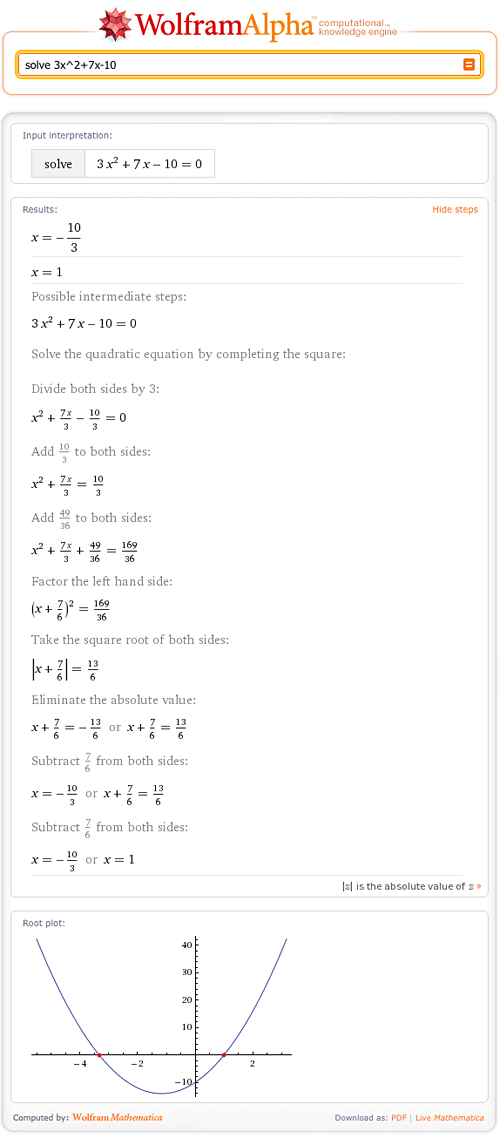You can find this widget, and many others, in the Wolfram|Alpha Widget Gallery. Customize or build your own to help you work through common math problems. Then add these widgets to your website or blog, and share them with friends on Facebook and other social networks.

Of course, Wolfram|Alpha also covers statistics and probability. For example, Wolfram|Alpha can compute coin tossing probabilities such as “probability of 21 coin tosses“, and provides information on normal distribution: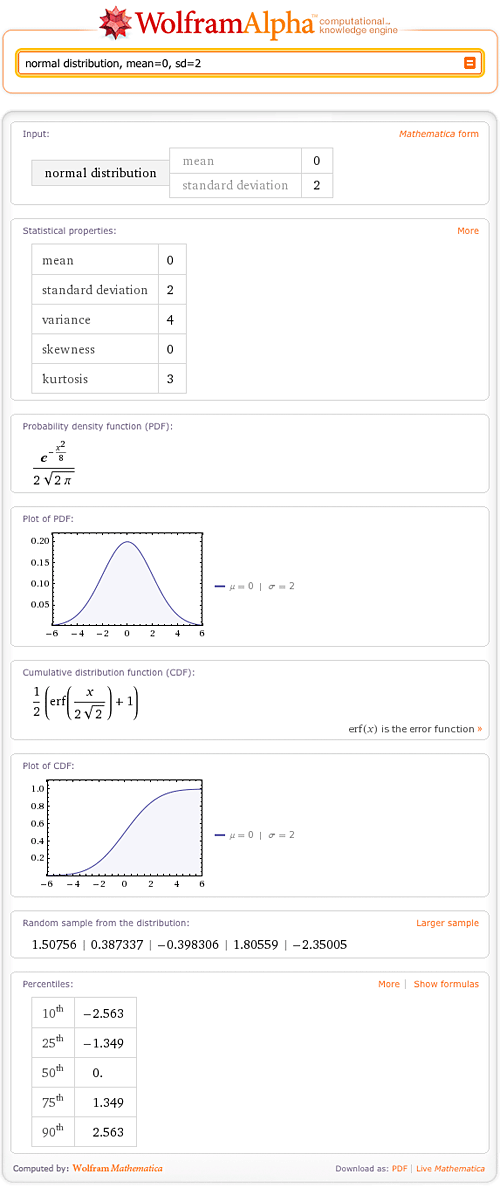Wolfram|Alpha also has the ability to locate and graph cusps and corners. Try “cusps of sqrt |x-2|” or  “corners of x + 2 |cos x|”.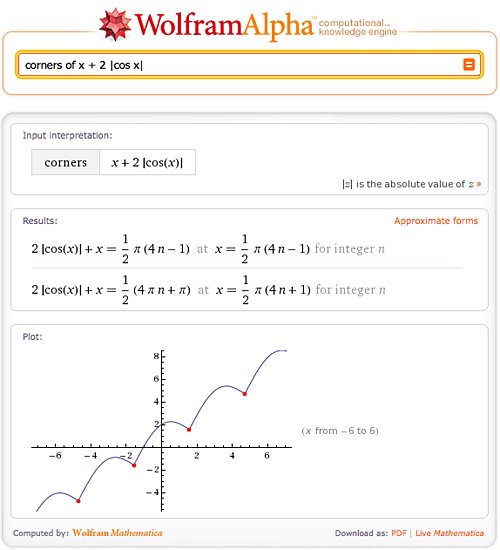Need to investigate stationary points? Enter “stationary points of (x^3-x^5+3x)^2” in Wolfram|Alpha. Or try “stationary point of cos t near t=2”.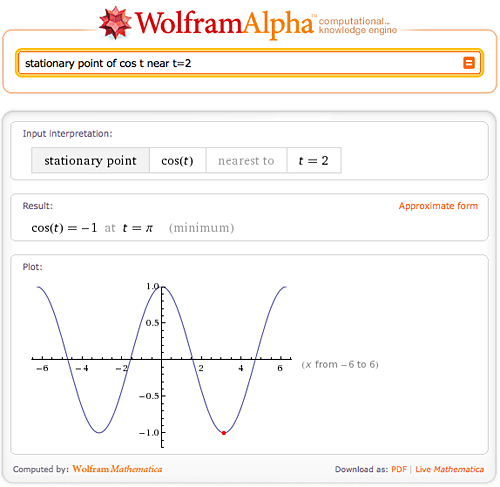Wolfram|Alpha can now calculate horizontal, vertical, oblique, and parabolic asymptotes. For example, try “oblique asymptotes of (x^3+7x+1)/(x^2-x+1)“: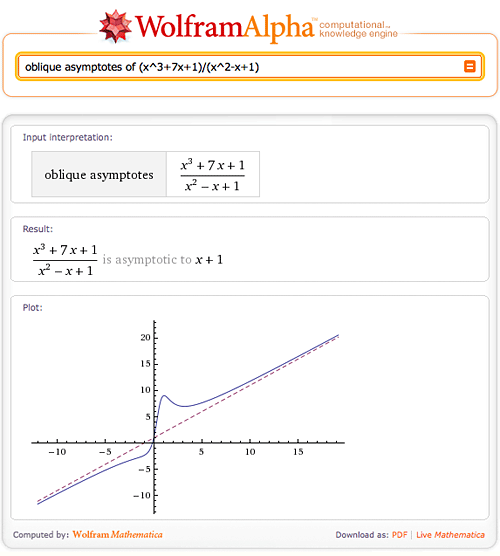More advanced mathematicians can find the gradient of a function or study Laplace Transforms.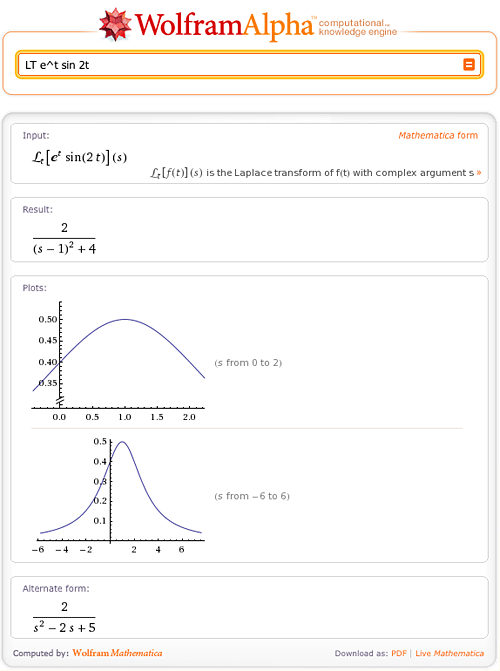Recently, Wolfram|Alpha expanded its coverage of geometry. For example, try querying information on a plane through 3 points: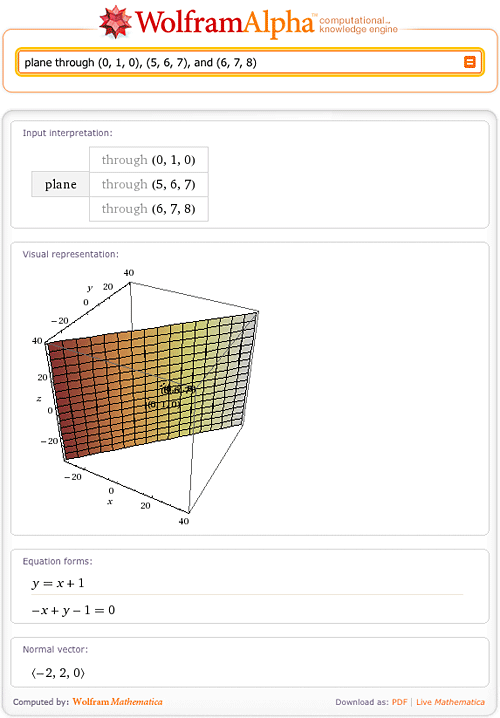Or a nine point center in a triangle given the vertices: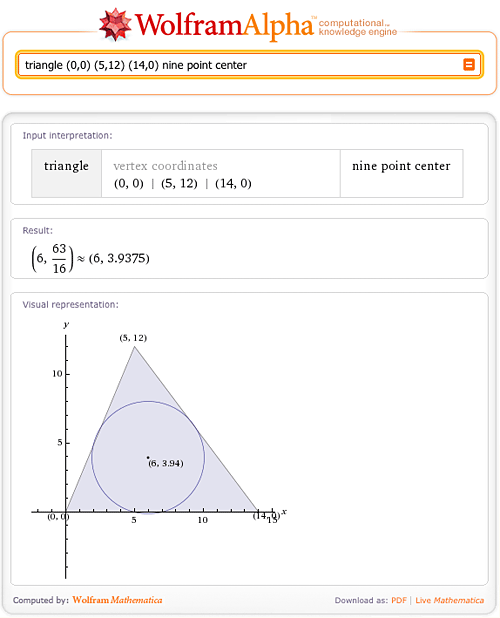Find the number of cells in a 7-cube:Wolfram|Alpha has also added the ability to evaluate PDFs.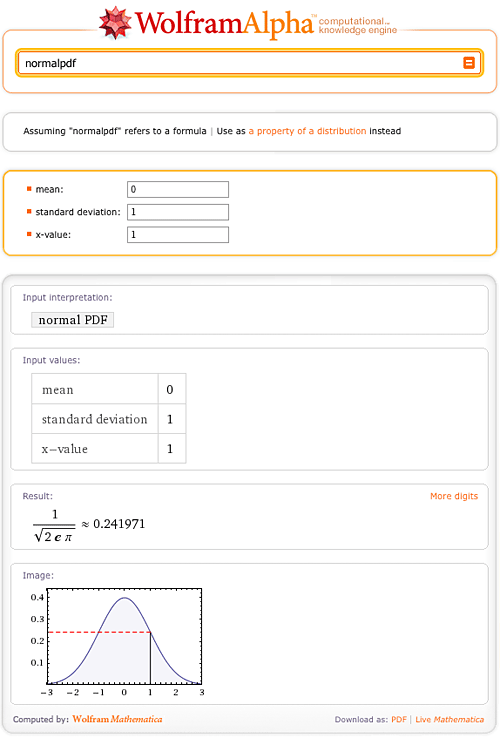As always, we will continue to add new mathematical features to Wolfram|Alpha and continue to update the existing content. The above examples are only a small fraction of Wolfram|Alpha’s abilities, so be sure to explore on your own.

Wolfram|Alpha is a great resource for both students and educators. It gives students the ability to get math help at home when their teachers are not available. And educators now have a new learning tool for the classroom, one which allows hands-on activities and exploration. So don’t forget to add Wolfram|Alpha and the Wolfram|Alpha app to your back-to-school list!

With such an ease of learning, I wish I am a student all over again!

Posted by Samuel Chen September 2, 2010 at 8:47 pm

You are still a student all your life. Forget this at your cost.

Posted by Brian Gilbert September 3, 2010 at 10:03 am

I consider the ‘Step by Step option in the output one of the most valuable features. It is hard for tutors to find the time for this for all their students in practice. It will give new hope to students who have been stuck on something.

Posted by Brian Gilbert September 3, 2010 at 10:07 am

AUTISM – my oldest son is high-functioning autistic and we’re going to work with his algebra teacher (he’s 13) on incorporating Wolfram Alpha into his learning program.
I’m encouraged by this development and will share it with our circle of friends.

Posted by Chris Moline, LEED AP September 3, 2010 at 10:19 am

Since I discovered this (trough TED talk)
It’s been a fascinating discovery what the capabilities are
of Wolfram| Alpha.. I LOVE IT !! ^^

For years I had a dream of finding a site or program that could solve equations
and many other stuff, like drawing graphs.

Keep Goin Forward Guys !

Posted by XavZz September 5, 2010 at 3:18 pm

Wolfram|Alpha has been an invaluable resource in my Calculus classes. I agree with Brian about the Step-by-Step, it is amazing that a computer can follow such human techniques.

Posted by reg.doug September 10, 2010 at 6:38 am

Marital Counselling and Wolfram Alpha – now W/A is helping my autistic son… humor me… let’s crowd source “Wiferam” Alpha 🙂 Imagine being able to factor in such marital conundrums as PMS, forgot to mow the lawn, not feeling beautiful… and know whether or not it’s ok to have a beer!
Just kidding, guys, Wolfram Alpha is a huge hit.
Keep up the great work.

Posted by Chris Moline LEED AP @webmindset September 13, 2010 at 6:57 pm